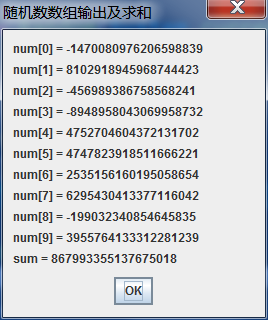• 本文实例讲述了Python生成随机数组的方法。分享给大家供大家参考，具体如下： 研究排序问题的时候常常需要生成随机数组来验证自己排序算法的正确性和性能，今天把Python生成随机数组的方法稍作总结，以备以后查看...
• 如下所示： ... 您可能感兴趣的文章:对python产生随机的二维数组实例详解Python生成随机数组的方法小结Python动态生成多维数组的方法示例Python使用random模块生成随机数操作实例详解numpy自动生成数组详解
• JS生成不重复的随机数组的简单实例 //获取数组中的随机数 //HF.Math.RandomNumbers是前缀，可以自己定义，主要看逻辑代码 HF.Math.RandomNumbers = function (startNum, endNum, count, repeat) { var ret = []; ...
• 今天小编就为大家分享一篇python生成多个只含0,1元素的随机数组或列表的实例，具有很好的参考价值，希望对大家有所帮助。一起跟随小编过来看看吧
• c语言学习资料，数据结构简单练习题，产生随机数并求亲自的最大值
•labview
• 1.numpy.random.rand()创建指定大小的随机数组，取值范围[0,1)importnumpy as np#创建2行2列取值范围为[0,1)的数组arr = np.random.rand(2,2)或importnumpy as np#创建一维数组，元素个数为10，取值范围为[0,1)arr1 ...

1.numpy.random.rand()

创建指定大小的随机数组，取值范围[0,1)

importnumpy as np#创建2行2列取值范围为[0,1)的数组

arr = np.random.rand(2,2)

importnumpy as np#创建一维数组，元素个数为10，取值范围为[0,1)

arr1 = np.random.rand(10)

2.numpy.random.randn()

创建指定大小的数组，取值范围为标准正态分布

#创建2行3列，取值范围为标准正态分布的数组

arr2 = np.random.randn(2,3)

3.numpy.random.randint()

创建指定大小的数组，数组数值随机取于[low,high)之间。high为空时则取[0,low)。需要用到size属性指定数组大小。

arr3 = np.random.randint(1,20,size=(2,2,3))

4.numpy.random.choice()

numpy.random.choice(a, size=None, replace=True, p=None)

a:指定的一维数组或者整数。如果是整数，则该方法等同于np.arange(a)

size:数组大小

replace:生成的数组中元素是否可以重复。默认为True，即可以重复

p:一维数组中每个元素出现的概率

5.numpy.random.shuffle()

将数组中的元素打乱。

arr4 = np.random.shuffle(arr1)

6.numpy.random.seed()

生成随机数种子

#生成一个随机数种子为seed1，参数可自己随意设定

np.random.seed(seed1)

它的作用是让下一次生成的随机数组与随机数种子关联，如果随机数组关联的随机数种子是一样的，且数组大小一样，则随机数组也是一样的。关联的做法就是在之前加上np.random.seed(seed1)。

简单地理解就是：在关联同一个随机数种子的前提下，第一次生成的数组其元素大小是随机的(当然是在指定的大小范围内)，其后生成的随机数组与第一次生成的数组的位置重叠部分，其元素是相同的，如果有扩展，则按随机原则生成。其本质是在随机前提下消除一定地随机性。

展开全文python随机数组
• 大部分程序和语言中的随机数，其实都只是伪随机。是由可确定的函数(常用线性同余)，通过一个种子(常用时钟)产生的。直观来想，计算机就是一种确定的、可预测的的...今天我们就来介绍一下如何在python生成随机数组。...大部分程序和语言中的随机数，其实都只是伪随机。是由可确定的函数(常用线性同余)，通过一个种子(常用时钟)产生的。直观来想，计算机就是一种确定的、可预测的的设备：一行行的代码是固定的，一步步的算法是固定的，一个个与非门是固定的。通过这些固定的东西自身产生真随机，当然不可能。程序和算法本身不能产生真随机，但是我们想办法可以迂回产生统计意义上的真随机。今天我们就来介绍一下如何在python生成随机数组。

方法：在python中，调用随机数模块的操作为import random。所以使用random.ranident,构造一个列表即可。

代码：import random

def random_list(start,stop,length):

if length>=0:

length=int(length)

start, stop = (int(start), int(stop)) if start <= stop else (int(stop), int(start))

random_list = []

for i in range(length):

random_list.append(random.randint(start, stop))

return random_list

如果知道了种子，或者已经产生的随机数，都可能获得接下来随机数序列的信息(可预测性)。我们使用random模块来操作正是使用了迂回手法来获取随机数组。更多学习推荐：python学习网。

展开全文• 众所周知，数组是一切数字的进阶版，随机数我们是了解了，可数组才是根本，下面小编就带大家看看随机数组的定义实现。例：随机生成10个数子，并放入一个数组，用消息框显示数组内容，再计算里数组元素的和，最后把...

众所周知，数组是一切数字的进阶版，随机数我们是了解了，可数组才是根本，下面小编就带大家看看随机数组的定义实现。

例：随机生成10个数子，并放入一个数组，用消息框显示数组内容，再计算里数组元素的和，最后把结果也显示在消息框中。

代码：import java.util.*;

import javax.swing.*;

public class SumRandom

{

public static void main(String[] args)

{

// TODO Auto-generated method stub

long num[] = new long; // 声明数组类型与大小；

String result = ""; // 存放数组内容输出结果，初值为空串

long sum = 0; // 和

Random in = new Random(System.currentTimeMillis()); // 创建Random类的对象引用in

for (int i = 0; i

{

// 求和并且保存输出结果到result

num[i] = in .nextLong(); // 给数组元素分配随机数

sum += num[i]; // 求和

result += "num[" + i + "] = " + num[i] + "\n"; // 存放数组内容输出结果

}

JOptionPane.showMessageDialog(null, result + "sum = " + sum, "随机数数组输出及求和", JOptionPane.CLOSED_OPTION);

// 创建消息框进行结果输出

}

}

结果：以上就是本文的所有相关内容，如果还有其他不懂的随机数常见问题的话就来奇Q工具网寻找答案吧。

推荐阅读

展开全文• python基础 - 生成随机数组

千次阅读 2020-06-14 22:51:22
python的random模块中有一些生成随机数字的方法，例如random.randint， random.random, random.uniform, random.randrange，这些函数大同小异，均是在返回指定范围内的一个整数或浮点数，下边简单解释一下这几个函数...

一、random模块

python的random模块中有一些生成随机数字的方法，例如random.randint， random.random, random.uniform, random.randrange，这些函数大同小异，均是在返回指定范围内的一个整数或浮点数，下边简单解释一下这几个函数。

from random import random
from random import randint
from random import uniform
from random import randrange

1、random.randint(low, hight)

参数：两个参数，必须是整数（或者小数位是0的浮点数），并且第一个参数必须不大于第二个参数。

返回：一个位于[low,hight]之间的整数。

print(random.randint(1, 10))      # 1
print(random.randint(1.0, 10.0))  # 10

2、random.random()

参数：无

返回：一个[0.0, 1.0)之间的浮点数

print(random.random())            # 0.6033317596268343

3、random.uniform(val1, val2)

参数：a, b

返回：两个数字区间的一个浮点数，[a, b) or [a, b] ，不要求val1小于等于val2

print(random.uniform(9.9, 2))     # 7.473451854502931

4、random.randrange(start, stop, step)

参数：以start开始，stop结束，step为步长

返回：列表中的随机整数，同样，三个参数均为整数（或者小数位为0），若start大于stop时 ，setp必须为负数.step不能是0.

print(random.randrange(1, 100, 2))     # 返回[1,100]之间的奇数
print(random.ranrange(100, 1, -2))     # 返回[100,1]之间的偶数

5、random.choice(seq)

返回seq序列中的任意一个元素。

seq = [1, 3, 5, 7, 9]
print([random.choice(seq) for _ in range(10)])  # [7, 9, 1, 7, 1, 1, 5, 9, 3, 5]

6、random.shuffle(seq)

类似洗牌，打乱顺序。

print(random.shuffle(seq))  # None
print(seq)  # [7, 5, 3, 9, 1]

7、random.sample(seq,n)

从序列中取n个随机的元素。

print(random.sample(seq, 4))  # [7, 3, 1, 9]

7、生成随机数组

（1）random.randint包装成随机数组函数

（2）用random_int_list函数来生成一个随机数组

def random_int_list(start, stop, length):
start, stop = (int(start), int(stop)) if start <= stop else (int(stop), int(start))
length = int(abs(length)) if length else 0
return [random.randint(start, stop) for _ in range(length)]

print(random_int_list(1, 100, 10))  # [2, 76, 29, 18, 23, 30, 7, 54, 3, 50]

二、使用numpy.random模块

1、np.random.rand()

生成[0.0, 1.0)之间的随机浮点数：

• 当没有参数时，返回一个随机浮点数；
• 当有一个参数时，返回该参数长度大小的一维随机浮点数数组。

参数建议是整数型，因为未来版本的numpy可能不支持非整形参数。

import numpy as np
print(type(np.random.rand(10)))  # <class 'numpy.ndarray'>
print(np.random.rand(10))
# [0.53024223 0.94762199 0.46473904 0.90486894 0.67282802 0.76911074 0.87628994 0.76377193 0.74619824 0.82050551]

该函数还可以用于生成多维数组：

print(np.random.rand(2, 4))  # 生成2行4列矩阵
# [[0.3499704  0.32819639 0.87229577 0.17463467] [0.62080204 0.1809523  0.20208164 0.65036285]]

2、np.random.randn

生成一个标准正态分布样本。

print(np.random.randn(10))
# [ 0.76265712  0.10098226 -0.20581081  0.74839501 -0.27727696 -0.96841736 1.55343188  0.09188352  0.54306779 -0.21720579]

3、np.random.randint(low[, high, size])

返回随机的整数，位于半开区间 [low, high)。

print(np.random.randint(10, size=10))  # [4 4 8 7 2 7 5 5 8 0]

4、random_integers(low[, high, size])

返回随机的整数，位于闭区间 [low, high]。

print(np.random.random_integers(10, size=10))  # [7 2 2 7 4 1 6 9 3 9]

5、np.random.shuffle(x)

类似洗牌，打乱顺序。

arr = np.arange(10)
print(np.random.shuffle(arr))  # None
print(arr)  # [5 7 8 0 3 4 6 9 1 2]

6、np.random.permutation(x)

参数：x 是 int or array_like

返回一个随机排列。

（1）If x is an integer, randomly permute np.arange(x).

print(np.random.permutation(10))  # [0 4 9 6 1 8 5 7 3 2]

（2）If x is an array, make a copy and shuffle the elements randomly.

print(np.random.permutation([0, 4, 9, 6, 1, 8, 5, 7, 3, 2]))  # [8 2 9 7 4 0 1 6 3 5]

arr = np.arange(9).reshape((3, 3))
print(arr)  # [[0 1 2] [3 4 5] [6 7 8]]
print(np.random.permutation(arr))  # [[6 7 8] [0 1 2] [3 4 5]]  只打乱行序

展开全文python random numpy
• 输入一个数字，生成10-99的一个随机数组，大小自定义生成随机数组
• 一、JAVA中生成随机数的方式1、在j2se中使用Math.random()令系统随机选取一个01之间的double类型小数，将其乘以一个数，比如25，就能得到一个025范围内的随机数，这个在j2me中没有2、在System类中有一个...java随机生成数组
• 有2中方式： static void Main(string[] args) { 起始: Stopwatch 总运行时间 = Stopwatch.StartNew(); ... var 随机数组 = Enumerable.Range(1, 22).Select(数 =&gt; new Random(G...
• C语言////产生随机数组

千次阅读 2020-06-05 20:04:04
最近在学习C语言数组，每次都要自己手输入一些测试数据，很是麻烦，因此查了一下怎么样产生随机数组，写法固定，源码如下 #include<stdio.h> //产生随机数，需要引入下面两个头文件 #include<stdlib.h>...指针 c语言
• js生成一个随机数组

千次阅读 2020-03-06 14:30:35
function creatArr(min, max) { var arr = []; for (var i = min; i ; i++) { arr.push(i) } ... //生成一个随机数组 var newArr = RandomArr(arr, spansLen) console.log(newArr)
• 获取随机数组（C#）

千次阅读 2020-03-02 22:45:45
在0到19的整数中获取十个不同的随机数组成一个随机数组 代码如下： int[] index = new int; for (int i = 0; i < 20; i++) index[i] = i; Random r = new Random(); //用来保存随机生成的不重复的10个数 int...C#
• C语言生成随机数和随机数组

万次阅读 多人点赞 2019-12-03 20:18:21
C语言生成随机数和随机数组 time函数要包含头文件<time.h> rand函数要包含头文件<stdlib.h> 随机数 int a; srand((unsigned)time(NULL)); // 播种 a = rand() % 200 + 1; 随机数组 #include <...C语言 随机数
• 代码如下:[removed] //从一个给定的数组arr中,随机返回num个不重复项 function getArrayItems(arr, num) { //新建一个数组,将传入的数组复制过来,用于运算,而不要直接操作传入的数组; var temp_array = new Array();...
• function getArrRandomly(arr) { var len = arr.length; //首先从最大的数开始遍历，之后递减 for(var i=len-1;i>=0;i--){ //随机索引值randomIndex是从0-arr.... //下面三句相当于把从数组随机抽取到的...
• 直接上代码，头文件代码 #ifndef SORT_SORTTOOLS_HPP #define SORT_SORTTOOLS_HPP #include <array> #include <random> #include <iostream> #include <iomanip> class SortTools { ...c++ 算法
• 本文实例讲述了javascript实现数组内值索引随机化及创建随机数组的方法。分享给大家供大家参考。具体如下： 今天在QW交流群里看到有同学讨论使数组随机化的问题，其中给出的算法很不错，让我想起了之前自己实现过的...
• rand：数组元素在0-1之间 randint：指定上下限的整数 ...demo.py（生成随机数组）： # coding=utf-8 import numpy as np # 生成随机数组(3行4列) (大小在0-1之间) t1 = np.random.rand(3, 4) ...Python numpy 随机数组
• 06_Numpy各种随机数组的生成方法 numpy.random模块中，有很多可以生成随机数的函数。 在此，对以下的内容进行说明： 生成均匀分布的随机数。 numpy.random.rand(): 0.0到1.0 numpy.random.random_sample(): 0.0到...python numpy
• 用Java写随机数组我做的项目用到的随机数组 我做的项目用到的随机数组 数组要求，主对角线为0，其余数组元素不为0。 效果图如下，有需求可自取，哇哈哈！ import java.util.Random; public class shuzu { public ...java html5...